# Zero-Dimensional Transport: The Continuously Stirred Reactor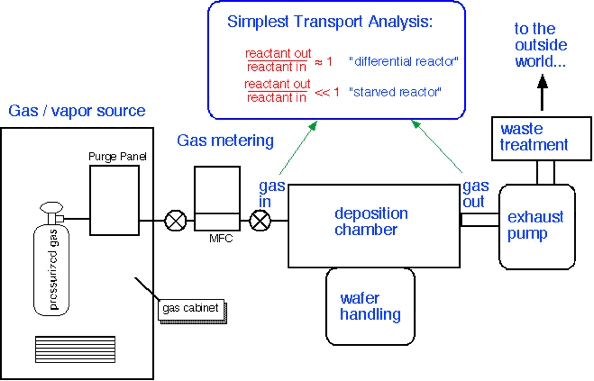A "differential" reactor consumes very little of the precursor which enters the chamber; concentrations are approximately constant throughout the reactor. On the other hand, a "starved" reactor uses most of the incoming precursor, and thus one can expect large gradients in concentration to exist throughout the reactor. Differential reactors are easy to understand and very useful in research. However, starved reactors are often encountered in industrial applications, because (as we'll see below) they are a consequence of rapid surface reaction rates often employed to obtain high throughput from the reactor.

How do we figure out what regime a reactor will operate in? If we know the geometry, gas flows and composition, and the speed of the reactions at the surface, we can make simple estimates of what is happening inside, using the assumption of a

"Zero-dimensional" reactor (simplest non-trivial transport analysis):
• concentrations are the same everywhere in the reactor (no gradients) => "zero-dimensional"
• often known in ChE literature as "continuously stirred reactor", CSTR approximation
• valid if diffusion or other mixing processes proceed much more rapidly than consumption and removal

Notation:

pre = precursor chemical for deposition
[pre] = concentration (moles/cm3, moles/m3, moles/liter, as convenient)

F = volume flow (e.g. m3/second)

Ks = surface reaction rate constant (units of velocity, e.g. cm/second or m/second)

ch = concentration in chamber
in = inlet concentration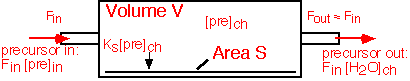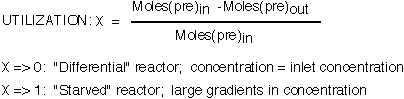To perform a detailed analysis, we balance stuff coming in, stuff going out, and stuff consumed at the surface: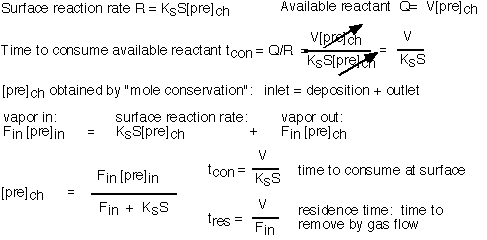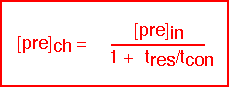The interesting limits are: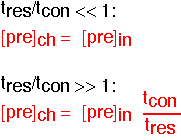The concentration of precursor in the reactor is essentially equal to the inlet concentration when the residence time is short compared to the time required to consume the species (i.e. conditions of high flow); this is the "differential" condition in which uniformity is likely to be good but efficiency of utilization is low. Since the consumption time is set by the surface reaction rate, differential conditions are likely for activated reactions at low temperatures, where the reaction rate is slow: this condition is often referred to as "surface limited".

The concentration of precursor is much less than the inlet concentration when the consumption time is fast (i.e. conditions of low flow or fast reaction rate). Here the uniformity of deposition is likely to be poor since large gradients in concentration have been created. This is the "starved" reactor condition; it is likely to occur for activated reactions at high temperatures, where the surface reaction rate is large, and is often known as "mass transport limited" deposition.

It is often the case that the surface limited or differential condition shows large temperature dependences, due to chemical reactions which require a significant activation energy. In the mass transport limited situation where the gas flows and transport dominate deposition rate, temperature dependence is weaker, since (as we'll see in studying transport) transport coefficients vary as nearly linear functions of temperature. Thus one often sees a diagram like :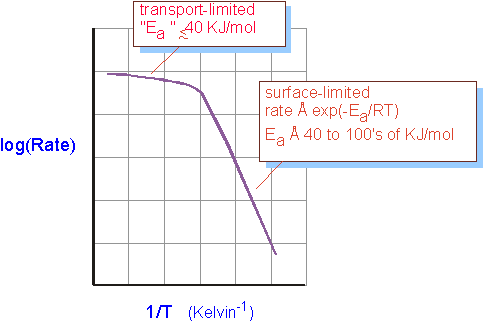However, it is well to be cautious in interpreting data in this fashion: small activation energies may simply indicate complex chemistry. Gradients across the reactor dependent on gas flows, as discussed above, are a more certain indicator of the reactor operating regime.

## Tutorial Speak with Forked Tongue

We started our treatment of zero-dimensional reactors assuming that concentration was uniform throughout the reactor; in the starved-reactor case we've just contradicted our initial assumption. This inconsistency is an indicator: we need to add something else to our treatment. To go further we can no longer ignore the mechanisms by which molecules move within the chamber: Mass Transport.